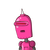# 1. Find the equations of tangent and normal to thecurve y = x⁴- 6x³ + 13x² -10x + 5 at (0,5).​

1. Find the equations of tangent and normal to the
curve y = x⁴- 6x³ + 13x² -10x + 5 at (0,5).​

### 1 thought on “1. Find the equations of tangent and normal to the<br />curve y = x⁴- 6x³ + 13x² -10x + 5 at (0,5).​”

1.At (0,5) slope of tangent is -10

Step-by-step explanation:

Equation of tangent is y-5=-10x

Slope of normal at (0,5) is 1/10

Equation of normal is 10y-50=x